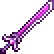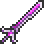•类型 \l!--\n --\g\{\{#vardefine:_typepage\!\{\{#or:\{\{l10n\!item_infobox\!typepage:@@@@\}\}\!\{\{tr\!\{\{ucfirst:@@@@\}\}\!link\ey\}\}\}\}\}\}\l!--\_\n --\g\{\{#vardefine:_typetext\!\{\{#or:\{\{l10n\!item_infobox\!type:@@@@\}\}\!\{\{tr\!\{\{ucfirst:@@@@\}\}\}\}\}\}\}\}\l!--\_\n --\g\{\{nowrap\!class\etag\!\{\{ifexists\!\{\{#var:_typepage\}\}\!\(\(\{\{#var:_typepage\}\}\!\{\{#var:_typetext\}\}\)\)\!\{\{#var:_typetext\}\}\}\}\}\}\l!--\n --\g 50 / 41 (近战) 6（普通击退力） 4% 25（快速度）253 需要 1 份

使用 \{\{#lstmap:\$soundfiles\$\!,\!%%%%\!\l!--\_print\_sounds\n --\g\lspan\_style\e"margin-left:2px;"\g\{\{sound\!\!\{\{trim\!%%%%\}\}\}\}\l/span\g\l!--\n --\g\!\}\}

!!Error: （请输入有效的值。参见Template:Modifier。）

## 制作

### 配方

• (12)

(\{\{#lstmap:\$soundfiles\$\!,\!%%%%\!\l!--\_print\_sounds\n --\g\lspan\_style\e"margin-left:2px;"\g\{\{sound\!\!\{\{trim\!%%%%\}\}\}\}\l/span\g\l!--\n --\g\!\}\})
• (10)
total: 2 row(s)

## 历史

• 电脑版 1.4.0.1
• 外观更新。
• 伤害由 47 提升至 50。
• 使用时间由 26 降低至 25。